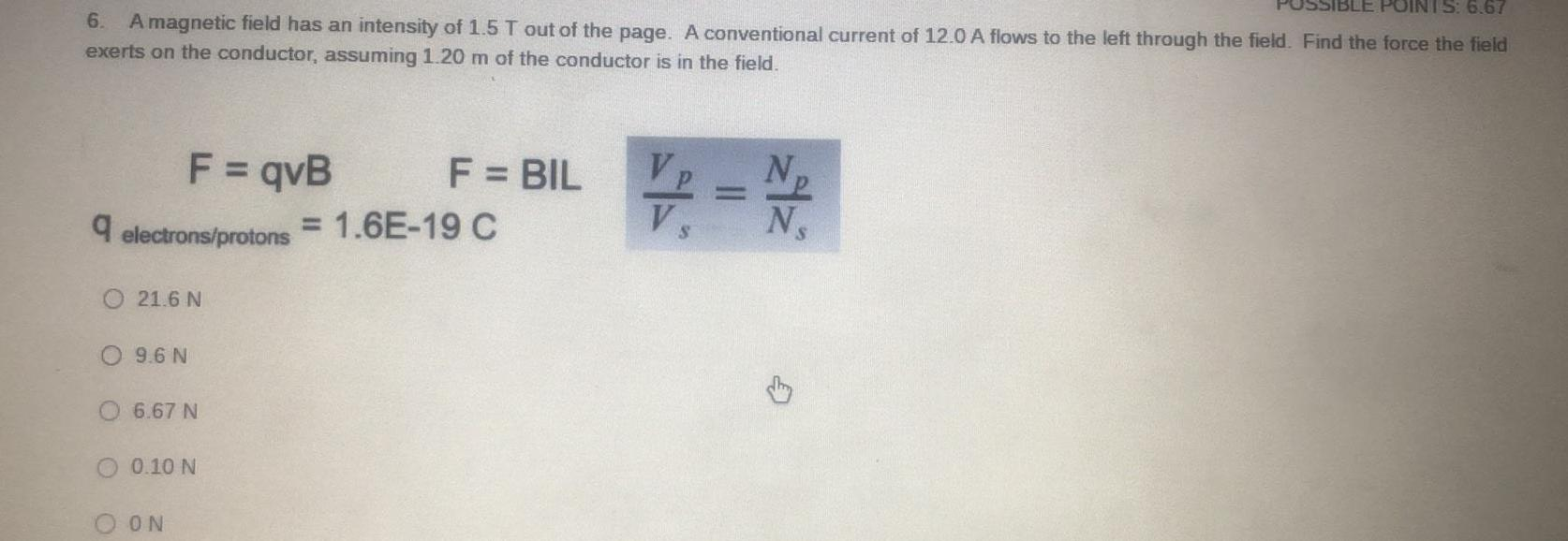Question:

# IS: 6.67 6. A magnetic field has an intensity of 1.5 T out

Last updated: 7/10/2022IS: 6.67 6. A magnetic field has an intensity of 1.5 T out of the page. A conventional current of 12.0 A flows to the left through the field. Find the force the field exerts on the conductor, assuming 1.20 m of the conductor is in the field. V=N/ q electrons/protons F = qvB 21.6 N 9.6 N 6.67 N 0.10 N ON F = BIL = 1.6E-19 C